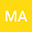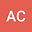loading page

Solving Fluid Dynamical Problem as Blasius Equation Using Variational Iteration Method With the Help of Padde Approximation Method
••• M. Tahmina Akter,
• A. Mansur Chowdhury
M. Tahmina Akter
Chittagong University of Engineering and Technology
Author ProfileA. Mansur Chowdhury
University of Chittagong
Author Profile## Abstract

Abstract : The Blasius equation is a well recognized third-order nonlinear ordinary differential equation which arises in certain boundary layer problems in the fluid dynamics. This article presents a way of applying He’s variational iteration method to solve the Blasius equation. Approximate analytical solution is derived with help of Padde approximate method and compared to the numerical result obtained from Adomian decomposition method.. Comparisons show that the present technique is precise and the using of He’s method does accelerate the convergence of the power series. And finally to see the behavior of that solution a robust and efficient algorithm is also programmed using Mathematica based on the present approach which can be easily employed to solve Blasius equation problems.